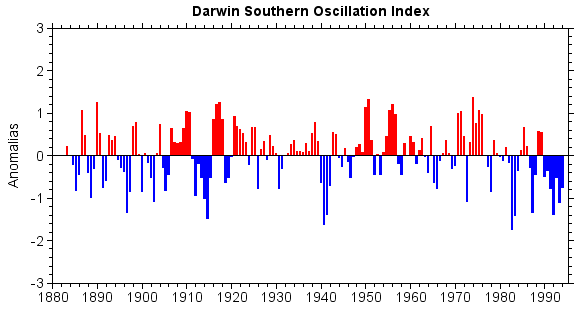# Bar chart¶

Bar chart was created by bar() function. The bar width in the chart was decided automatically according to data series number.

menMeans = [20, 35, 30, 35, 27]
n = len(menMeans)
ind = arange(n)
width = 0.2
bar(ind, menMeans, width, color='r', label='Men')
womenMeans = [25, 32, 34, 20, 25]
bar(ind + width, womenMeans, width, color='y', label='Women')
xlim(-0.2, 4.6)
ylim(0, 40)
ylabel('Mean age')
xticks(ind + width, ['G1','G2','G3','G4','G5'])
legend()
title('Bar chart example')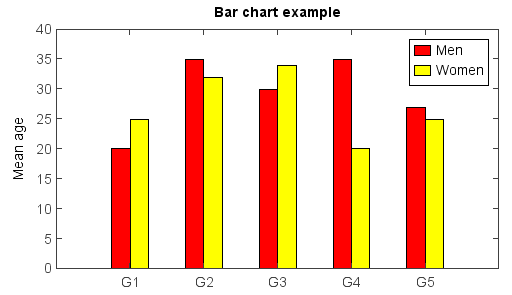The bar width and plot position could be set manually with x array and width argument.

menMeans = [20, 35, 30, 35, 27]
n = len(menMeans)
ind = arange(n)
width = 0.35
gap = 0.06
bar(ind, menMeans, width, color='r', label='Men')

womenMeans = [25, 32, 34, 20, 25]
bar(ind + width + gap, womenMeans, width, color='y', label='Women')

xlim(-0.2, 5)
ylim(0, 40)
ylabel('Mean age')
xticks(ind + width + gap / 2, ['G1','G2','G3','G4','G5'])
legend()
title('Bar chart example')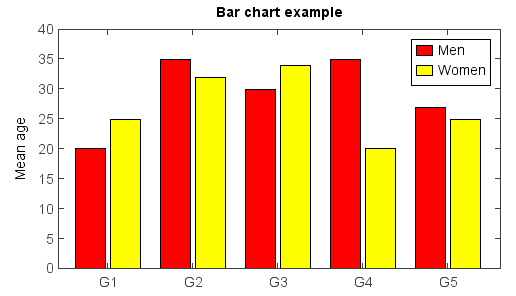Y error bar and text labels on bars:

menMeans = [20, 35, 30, 35, 27]
std_men = (2, 3, 4, 1, 2)
n = len(menMeans)
ind = arange(n)
width = 0.35
gap = 0.06
bar(ind, menMeans, width, yerr=std_men, color='r', ecolor='b', label='Men')
for j in range(n):
text(ind[j] + width / 4, menMeans[j] + 2, str(menMeans[j]))

womenMeans = [25, 32, 34, 20, 25]
std_women = (3, 5, 2, 3, 3)
bar(ind + width + gap, womenMeans, width, yerr=std_women, color='y', ecolor='g', label='Women')
for j in range(n):
text(ind[j] + + width + gap + width / 4, womenMeans[j] + 2, str(womenMeans[j]))

xlim(-0.2, 5)
ylim(0, 40)
ylabel('Scores')
xticks(ind + width + gap / 2, ['G1','G2','G3','G4','G5'])
legend()
title('Scores by group and gender')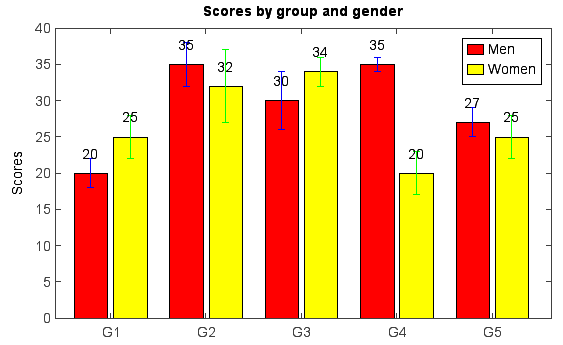Stacked bar example using bottom argument:

menMeans = [20, 35, 30, 35, 27]
n = len(menMeans)
ind = arange(n)
width = 0.35
bar(ind, menMeans, width, color='r', ecolor='b', label='Men')

womenMeans = [25, 32, 34, 20, 25]
bar(ind, womenMeans, width, bottom=menMeans, color='y', ecolor='g', label='Women')

xlim(-0.2, 5)
ylim(0, 80)
ylabel('Scores')
xticks(ind + width / 2, ['G1','G2','G3','G4','G5'])
legend()
title('Scores by group and gender')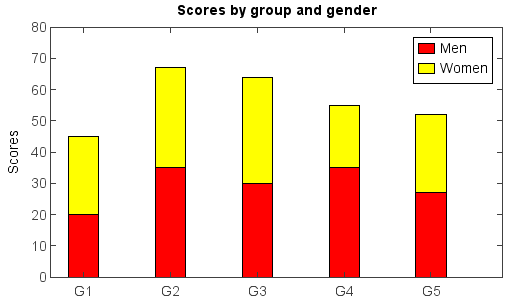Hatch fill example using hatch argument:

menMeans = [20, 35, 30, 35, 27]
std_men = (2, 3, 4, 1, 2)
bar(menMeans, yerr=std_men, color='r', label='Men', hatch='-')

womenMeans = [25, 32, 34, 20, 25]
std_women = (3, 5, 2, 3, 3)
bar(womenMeans, yerr=std_women, color='b', label='Women', hatch='\\')

ylim(0, 40)
ylabel('Mean age')
xticks(arange(1, len(menMeans) + 1), ['G1','G2','G3','G4','G5'])
legend()
title('Bar chart example - Hatch')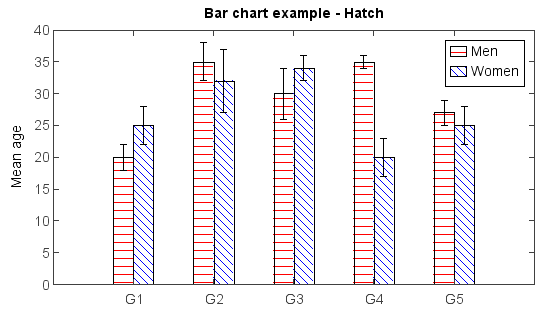An example to draw Darwin Southern Oscillation Index with bar chart:

fn = 'D:/Temp/nc/soi.nc'
yms = f['date'][::8]       #Year and month
dsoik = f['DSOI_KET'][::8] #Darwin SOI Index via KET 11pt Smth
dsoid = f['DSOI_DEC'][::8] #Darwin Decadal SOI Index

#Set dates and colors
dates = []
cols = []
for ym,d in zip(yms,dsoik):
dates.append(datetime.datetime(ym / 100, ym % 100, 1))
if d >= 0:
cols.append('r')
else:
cols.append('b')

#Bar plot
bar(dates, dsoik, color=cols, edgecolor=None)
xlim(dates, dates[-1])
ylim(-3, 3)
xaxis(axistype='time', minortick=True, tickin=False)
yaxis(minortick=True, tickin=False)
ylabel('Anomalias')
title('Darwin Southern Oscillation Index')